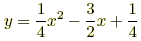index: click on a letter A B C D E F G H I J K L M N O P Q R S T U V W X Y Z A to Z index index: subject areas numbers & symbols sets, logic, proofs geometry algebra trigonometry advanced algebra & pre-calculus calculus advanced topics probability & statistics real world applications multimedia entrieswww.mathwords.com about mathwords website feedback

Focus of a Parabola

The focus of a parabola is a fixed point on the interior of a parabola used in the formal definition of the curve.

A parabola is defined as follows: For a given point, called the focus, and a given line not through the focus, called the directrix, a parabola is the locus of points such that the distance to the focus equals the distance to the directrix.

Note: For a parabolic mirror, all rays of light emitting from the focus reflect off the parabola and travel parallel to each other (parallel to the axis of symmetry as well).Example:This is a graph of the parabolawith all its major features labeled: axis of symmetry, focus, vertex, and directrix.